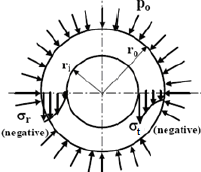Courses

# Thin Cylinders & Thick Cylinders - MCQ Test 1

## 20 Questions MCQ Test RRB JE for Mechanical Engineering | Thin Cylinders & Thick Cylinders - MCQ Test 1

Description
This mock test of Thin Cylinders & Thick Cylinders - MCQ Test 1 for Mechanical Engineering helps you for every Mechanical Engineering entrance exam. This contains 20 Multiple Choice Questions for Mechanical Engineering Thin Cylinders & Thick Cylinders - MCQ Test 1 (mcq) to study with solutions a complete question bank. The solved questions answers in this Thin Cylinders & Thick Cylinders - MCQ Test 1 quiz give you a good mix of easy questions and tough questions. Mechanical Engineering students definitely take this Thin Cylinders & Thick Cylinders - MCQ Test 1 exercise for a better result in the exam. You can find other Thin Cylinders & Thick Cylinders - MCQ Test 1 extra questions, long questions & short questions for Mechanical Engineering on EduRev as well by searching above.
QUESTION: 1

### A thin cylinder of inner radius 500 mm and thickness 10 mm is subjected to an internal pressure of 5 MPa. The average circumferential (hoop) stress in MPa isb

Solution:

Inner radius (r) = 500 mm
Thickness (t) = 10 mm
Internal pressure (p) = 5 MPa

Hoop stress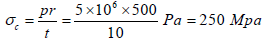QUESTION: 2

Solution:
QUESTION: 3

### A cylindrical container of radius R = 1 m, wall thickness 1 mm is filled with water up to a depth of 2 m and suspended along its upper rim. The density of water is 1000 kg/m3 and acceleration due to gravity is 10 m/s2. The self-weight of the cylinder is negligible. The formula for hoop stress in a thin-walled cylinder can be used at all points along the height of the cylindrical container.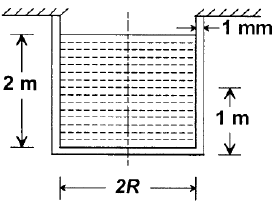The axial and circumferential stress (σa ,σc ) experienced by the cylinder wall at middepth (1 m as shown) are

Solution: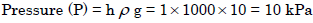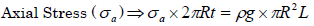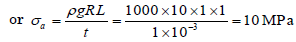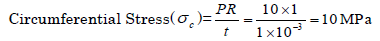QUESTION: 4

Match List-I with List-II and select the correct answer: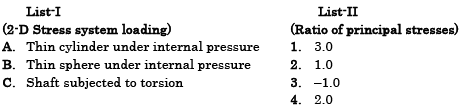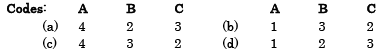Solution:
QUESTION: 5

A thin cylindrical shell is subjected to internal pressure p. The Poisson's ratio of the material of the shell is 0.3. Due to internal pressure, the shell is subjected to circumferential strain and axial strain. The ratio of circumferential strain to axial strain is:

Solution:

Circumferential strain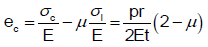Longitudinal strain,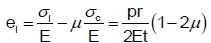QUESTION: 6

When a thin cylinder of diameter 'd' and thickness 't' is pressurized with an internal  pressure of 'p',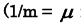is the Poisson's ratio and E is the modulus of elasticity), then

Solution:

Ratio of longitudinal strain to circumferential strain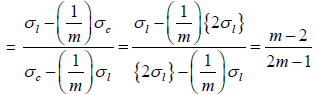Circumferential strain,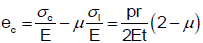Longitudinal strain,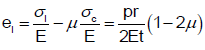QUESTION: 7

A thin cylinder with both ends closed is subjected to internal pressure p. The longitudinal stress at the surface has been calculated as σo. Maximum shear stress at the surface will be equal to:

Solution: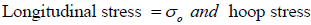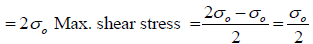QUESTION: 8

A penstock pipe of 10m diameter carries water under a pressure head of 100 m. If the wall thickness is 9 mm, what is the tensile stress in the pipe wall in MPa?

Solution:

Tensile stress in the pipe wall = Circumferential stress in pipe wall = Pd/2t

Where,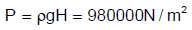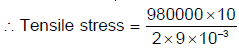QUESTION: 9

A seamless pipe of diameter d m is to carry fluid under a pressure of p kN/cm2. The necessary thickness t of metal in cm, if the maximum stress is not to exceed σ kN/cm2, is

Solution:
QUESTION: 10

In strain gauge dynamometers, the use of how many active gauge makes the dynamometer more effective?

Solution:
QUESTION: 11

The volumetric strain in case of a thin cylindrical shell of diameter d, thickness t, subjected to internal pressure p is:

(Where E = Modulus of elasticity, μ = Poisson's ratio for the shell material)

Solution:
QUESTION: 12

From design point of view, spherical pressure vessels are preferred over cylindrical pressure vessels because they

Solution:
QUESTION: 13

A thin walled water pipe carries water under a pressure of 2 N/mm2 and discharges water into a tank. Diameter of the pipe is 25 mm and thickness is 2·5 mm. What is the longitudinal stress induced in the pipe?

Solution: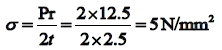QUESTION: 14

Assertion (A): A thin cylindrical shell is subjected to internal fluid pressure that induces a 2-D stress state in the material along the longitudinal and circumferential directions.

Reason(R): The circumferential stress in the thin cylindrical shell is two times the magnitude of longitudinal stress.

Solution:

For thin cell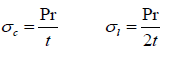QUESTION: 15

Assertion (A): For a thin cylinder under internal pressure, At least three strain gauges is needed to know the stress state completely at any point on the shell.

Reason (R): If the principal stresses directions are not know, the minimum number of strain gauges needed is three in a biaxial field.

Solution:

For thin cylinder, variation of radial strain is zero. So only circumferential and longitudinal strain has to measurer so only two strain gauges are needed.

QUESTION: 16

Circumferential and longitudinal strains in a cylindrical boiler under internal steam pressure are  ε1 and εrespectively. Change in volume of the boiler cylinder per unit volume will be:

Solution:

Volumetric stream = 2 x circumferential strain + longitudinal strain.

QUESTION: 17

A thin cylinder of diameter „d‟ and thickness 't' is subjected to an internal pressure 'p' the change in diameter is (where E is the modulus of elasticity and μ is the Poisson's ratio)

Solution:
QUESTION: 18

A round bar of length l , elastic modulus E and Poisson's ratio μ is subjected to an ax ial pull 'P'. What would be the change in volume of the bar?

Solution: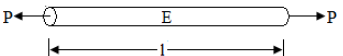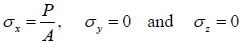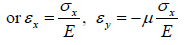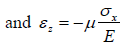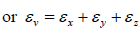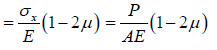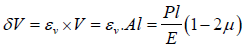QUESTION: 19

A thick cylinder is subjected to an internal pressure of 60 MPa. If the hoop stress on the outer surface is 150 MPa, then the hoop stress on the internal surface is:

Solution:

If internal pressure = pi; External pressure = zero

Circumferential or hoop stress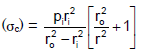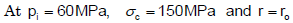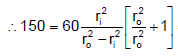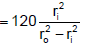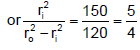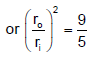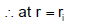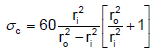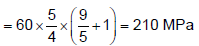QUESTION: 20

Where does the maximum hoop stress in a thick cylinder under external pre ssure occur?

Solution:

Circumferential or hoop stress = σt